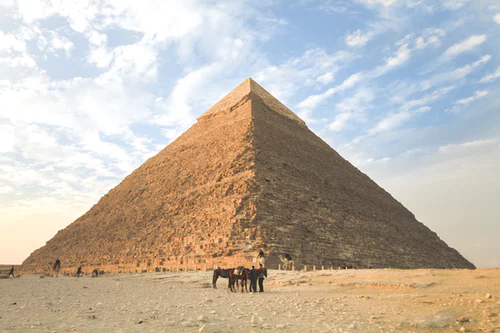# Formulae

## Understanding and using mathematical formulae

Formulae are what we use to solve a specific problem. For the higher paper you will need to learn and remember a LOT of formulas.

Checkout the Pearson website for a beautiful poster of all the formulas you’ll need to remember!

## Rearranging formulae

To use a formula you will need to substitute in variables you have. Sometimes you may have to rearrange formula. To do this you need to remember the inverse of operations.

For example, if we want to find the height of a pyramid where we are given the area of the base and the volume. Firstly we need to remember the formula to find the volume of a pyramid: ⅓ x area of the base x height = volume.

Now we need to think how we can use the inverse operations to make our formula. Height =……

We need to think about how the other variables are connect to the height, in this case they are multiplied. The inverse of multiplication is divide. Therefore we will need to divide the volume by ⅓ x area of the base.Height = Volume__ /__ ⅓ x area of the base

Once we have rearranged the formula we can substitute in our variables and find our answer.

## Algebra as functions

Thinking through what happens to each expression or equation enables us to rearrange equations more easily.

This idea of Input ____ Function __→ ____Output __is something that we write algebraically as f(x).

A function can be very simple, such as: f(x)= 2x. In this case we will multiply x by 2

Functions can also be more complicated such as: f(x)= 2x2 + 6. In this case we need to square x, double it and then add 6.

There might be occasions when you will need to show the results for a certain number of values. For example, what are the outputs for the function f(x) = 4x + 3 between 2<x<5

x = 3

f(3)= 4(3) + 3

= 15

x = 4

f(4) = 4(4) + 3

= 19

The area of a circle is 10 cm, what is the diameter of the circle to 2 decimal places?